# An Intuitive Guide To Statistical Significance

## An Intuitive Guide To Statistical Significance

The purpose of this blog post is to provide an intuitive understanding of why statistical significance works the way it does.  The most important concept for that is to understand why small differences in measured results become more statistically significant as you get more measurements. And the reason for this is that the standard deviation of the mean decreases significantly as you get more measurements, even if the standard deviation of the population doesn’t change at all.

There are 5 main types of statistical significance tests.  They are

• Z Test
• 1 Sample T-Test
• Paired T-Test
• 2 Sample T-Test with Equal Variance
• 2 Sample T-Test with Unequal Variance

This blog post does not walk through all the examples and equations for each of the types.  This post gives the overarching framework that ties them all together.  If you want to see more detail about each type of T-Test you might be interested in my book  “Hypothesis Testing: A Visual Introduction To Statistical Significance

And if you want all the different equations and a quick example of when you would use each on a single page, check out this cheat sheet for hypothesis testing.  http://www.fairlynerdy.com/hypothesis-testing-cheat-sheets/This hypothesis testing summary post here a summary of some of the other key points for statistical significance not covered on this page

## What Is Statistical Significance?

When you are testing for statistical significance, the equation you are asking is, how unlikely would this outcome have been if it just happened by random chance?

You are likely aware that most real life events have some degree of scatter in the results.  If you take a bunch of measurements for similar things you will get some measurements that are high, some that are low, and some that fall in the middle.  For instance, if you are buying a prepackaged 5 lbs. bag of apples at the grocery store, and you weigh all 20 bags of apples that are on the shelf at the time, you will likely see some that are around 5 lbs., some that are 5.1 lbs, some that are 5.2 lbs, and maybe even a couple that are 4.9 lbs.

You might come in one day and measure 10 bags of apples and find that the average weight of those 10 bags is 5.2 lbs.  You might come in a couple of months later and measure 10 different bags and find that the average weight of those bags is 5.0 lbs.  Does that change in results mean that something changed to cause the new bags to be lighter?  For instance, maybe the apple packers are using more accurate machines to make sure all the weights are barely above the guaranteed 5 lbs.  Or maybe that change in results is just a random outcome of the particular bags you chose to measure on that particular day.   Statistical Significance is a way of determining which of those possibilities is true.

What we are doing with statistical significance calculations is determining how unlikely an outcome was to occur by random chance, and then deciding if that probability is unlikely enough that we can conclude something other than random chance caused that outcome.

The two most important things in a statistical significance calculation are the distance the average value of your measured data is from what you are comparing it against, and the standard deviation of what you are measuring.  It is easy to understand how the difference in measurements is important.  For instance, in the apple example, if my first group of apples weigh 7 lbs. and the second group weigh 5.0 lbs. there is more likely to be a significant difference than if my first group weigh 5.2 lbs. and my second group weigh 5.1 lbs.  The greater the difference in measurements, the greater the significance, assuming that all other values are equal.

What Is The Standard Deviation

Standard deviation is the second important topic in calculating statistical significance.  It is worth going over how the standard deviation works.  Standard deviation is a way of measuring how spread out your measured values are. If the values you are measuring are all clustered together, they will have a low standard deviation.  If they have a lot of variation in the measurements, they will have a high standard deviation.

For example, if you had measured 5 bags of apples and their weights were 5.0, 5.1, 5.1, 5.2, and 5.2 lbs, those results are a lot more tightly clustered, and hence lower standard deviation, than if you had measured 5 bags and gotten weights of 5.0, 5.6, 5.9, 6.2, 6.5 lbs.

The image below shows what we typically think of when we think about standard deviation.  There is a mean value at the center of a normal curve.   If you make another measurement of the same type of data it will fall somewhere on that normal curve, with decreasing likelihood the farther you get away from the mean value.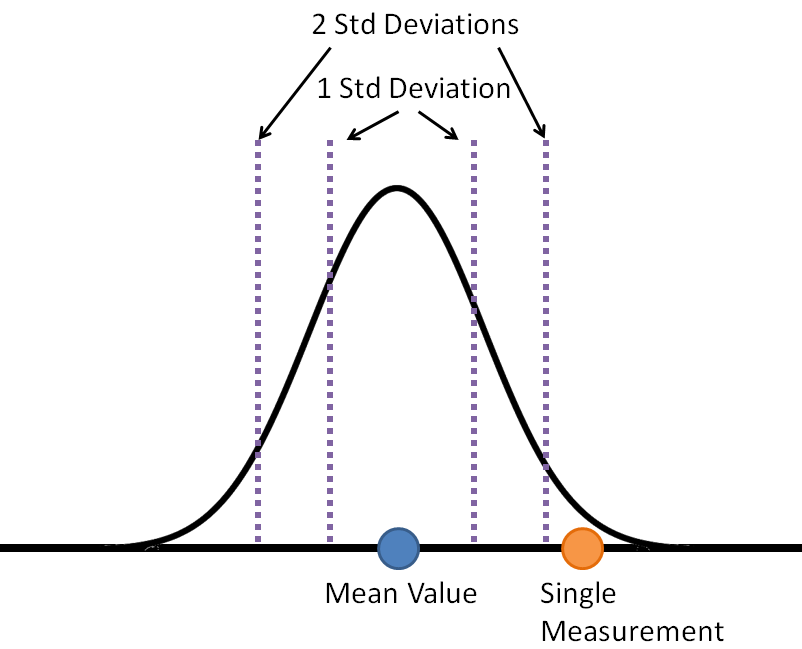With a typical normal curve

• 68 percent of the data will fall within 1 standard deviation of the mean
• 95 percent of the data be within 2 standard deviations
• 99.7 percent of the data is within 3 standard deviations

So if we know the mean value and standard deviation of our data, we know the distribution of results that we expect to get.  We know that if we get a result that is 2 standard deviations away from the mean, that it is a rare event because 95% of the results will fall within 2 standard deviations of the mean.

With hypothesis testing, what we are doing is turning that chart around and asking the question in reverse.  Now what we are doing is putting a normal curve around our measured data, and asking the question “How likely is it that this measured data came from the same source as the reference data?”  We are asking how many standard deviations the reference value is from our mean value.   This is shown in the chart below.Now, this might seem like it was a useless distinction.  If the reference value was two standard deviations away from the measured value, then the measured value will be two standard deviations away from the reference value.   That is completely true, but only if we only have a single piece of measured data.

## Statistical Significance Becomes Important When You Have More Than 1 Measurement

The one piece of information that you can’t figure out just using a typical normal curve is what to do with additional measurements.   Let’s say that you have a mean and standard deviation for a set of data. For instance, maybe the bags of apples on week weigh 5.2 lbs on average and have a standard deviation of 0.2 lbs.  If you go back to the store a month later and weigh a bag of apples that is 2 standard deviations heavier, at 5.6 lbs. you will think that is unusual because a 2 standard deviation difference is a rare event.  But what if your next measurement is 5.4 lbs, and your next one after that is 5.1 lbs.?  Do the additional measurements make you more or less convinced that the new bags of apples are different than the old bags?

The effect of increased quantity of measurements is the single most important concept in understanding statistical significance.  If you understand this, then you understand statistical significance. The rest of it is just knowing when to apply which equation.

The concept is this: We do not care about the standard deviation of our data. What we care about is the standard deviation of the mean value of all of our measurements. And that standard deviation of the average can change as you make additional measurements.

This is shown in the chart below. Like the chart above, it has a normal curve centered on the mean values of the measured data. However, due to the fact that there are more measurements in this data, rather than just the single measurement in the chart above, this normal curve is narrowerSince the normal curve is narrower, the reference value falls farther away from the measured average, in terms of the number of standard deviations.  As a result, we will conclude that it is less likely that our measured values and the reference value came from the same set of data.  I.e. we are less likely to get a 4 standard deviation difference than a 2 standard deviation difference unless there is an actual change that drove the difference (i.e. we are using a different group of people to pack the bags of apples this week, and they are packing them heavier.)

## Why Does The Standard Deviation Of The Mean Decrease With More Measurements?

We stated that what we are interested in is the standard deviation of the mean value of all of the measurements we make.  The standard deviation of that average value decreases as you increase the number of measurements made.  Why is that? Fortunately, we don’t have to use complicated math to explain this topic.  You have almost certainly seen it before, although you probably didn’t think about it in these terms.

The way to understand the standard deviation of the average result is to think about what happens when you roll a single die vs what happens when you roll 2 die or more dice.

If you roll a single die, you are equally likely to get a 1, 2, 3, 4, 5, or 6.  Each value will come up one-sixth of the time, so the probability distribution of a single die rolled six times will have each value coming up one time.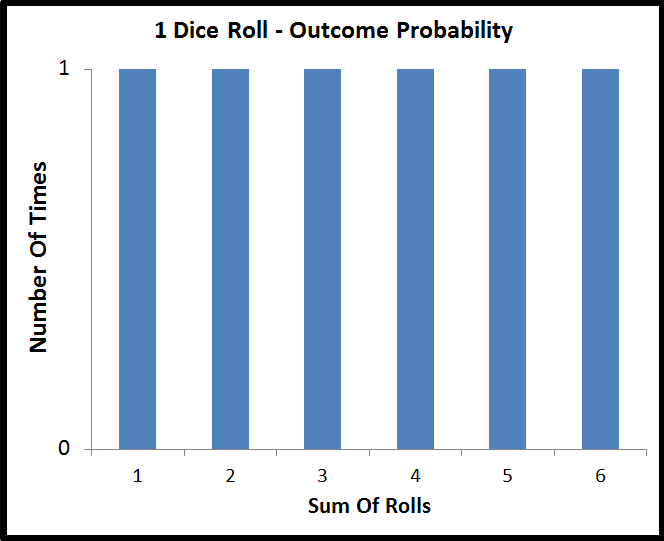There is no way to predict the outcome of a single die roll.   No outcome is more likely than any other.

Now, what happens if you roll two dice, and add their values? Well, there are 36 different permutations of die rolls that you can get out of two dice, 6 values from the first die, multiplied by 6 values from the second die.  However, there are only 11 different sums that you can get from those two dice, the values of 2 through 12.

Those 36 different die permutations don’t map evenly onto the 11 different possible sums. You are more likely to get values in the middle of the range than values on the edges. The probability distribution of the sum of two dice is shown below.All of a sudden, you can start to make predictions on the outcome of rolling 2 dice, even though you can’t do it for any specific die.  The single most likely sum is a 7, which is why casinos make that value a number that they win on in the game of craps.  This effect where the sum of two dice have an unequal probability distribution also shows up in the gameplay of a lot of board games, for instance, it is the key mechanic in the game “Settlers Of CatanFor our purposes, the key point is that the probability of outcomes is more concentrated in the center of the graph for two die rolls than it is for a single die roll. That concentration of probability into the center of the graph doesn’t stop with 2 dice.  If you sum the value of 3 dice there are 216 different permutations of rolls (6 * 6 * 6) mapped onto 16 possible values (3-18).   The probability distribution for 3 dice summed is shown below.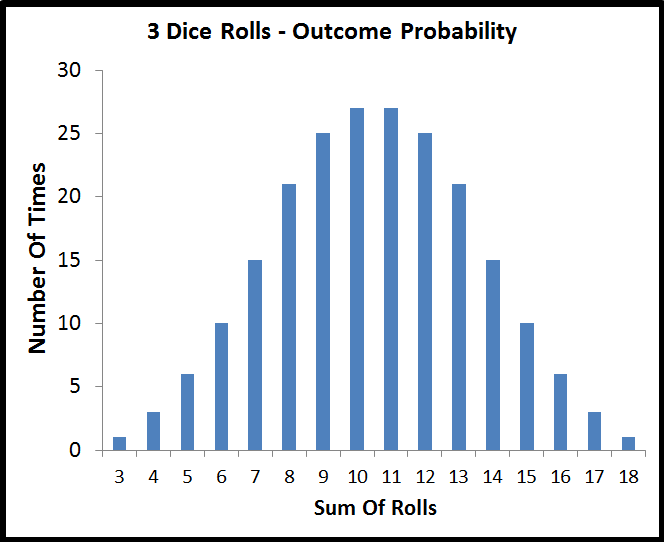Even though it isn’t quite as visually obvious as going from 1 die to 2 dice, summing 3 dice has a greater concentration of probability in the center of the graph than the sum of 2 dice. That process would continue if we kept rolling additional dice.

### The Probability Distribution Of The Average Result

So far with these dice, we’ve talked about the sum of the dice values.   Now let’s talk about the average value.  To calculate the average value, just take the sum and divide by the number of dice.  So for instance, if you rolled a sum of 7 on 2 dice, then your average value was 3.5.

The probability distribution of the average value for 1, 2, and 3 dice rolled is shown in the plot below.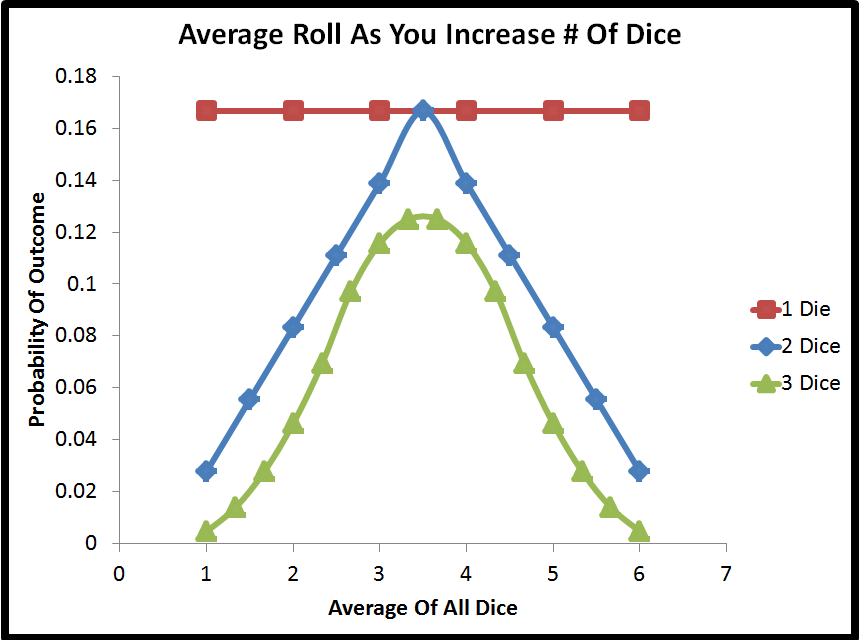This plot makes a few things obvious

• Not matter how many dice are rolled, the mean value is always centered on 3.5. This is because the average of all the numbers on a single die, (average of 1, 2, 3, 4, 5, 6) is 3.5
• As you increase the number of dice, the probability of getting an average value that is on the edges of the range decreases dramatically. The bulk of the probability shifts to the center of the graph

## Why Is This Important For Statistical Significance?

Let’s pause for a second and reiterate why we care about the above result, in terms of statistical significance.  What we see is that by making multiple measurements, we can be more confident in their average value than we can in the result of any single measurement.  The range of possible outcomes for the average of all measurements is the same as the range of possible outcomes for a single measurement, but the distribution over that range is a lot more clustered in the center.  What we are seeing is that the standard deviation of the mean of multiple measurements is lower than the standard deviation of a single measurement.   Since statistical significance is about the number of standard deviations outside of the mean value your results are, this is very important.

## Calculating The Standard Deviation Of The Average Result

In this plotIt might be surprising that the probability for every single possible average for 3 dice is lower than their counterpart for 2 dice, and also for 1 die. That is because as you increase the number of dice, the probability is spread among more possible outcomes. There are only 6 possible outcomes with 1 die, but 11 possible outcomes with 2 die and 16 possible outcomes with 3 die.   In order to get consistent probability distributions with different numbers of dice, we can use a histogram and ‘binning’ to make sure the probability is spread among the same number of outcomes.

That wouldn’t plot very well for 1, 2 and 3 dice; however here is a binned result of the probability distribution for the average roll when rolling 5, 10, and 20 dice.As you can see, the greater the number of rolls, the more the probability distribution of the average value is clustered around the average value of 3.5 and less at the edges. This means that the standard deviation of the average is decreasing as you increase the number of samples used to calculate it.

We can, in fact, calculate the standard deviation of the average for a given number of dice.  For a single die, this is just the population standard deviation of [1, 2, 3, 4, 5, 6], which is 1.7078.   For two dice, it would be the population standard deviation of the 36 possible average values (11 distinct values) of [1, 1.5, 1.5, 2, 2, 2, 2.5, 2.5, 2.5, 2.5, 3, 3, 3, 3, 3, 3.5, 3.5, 3.5, 3.5, 3.5, 3.5, 4, 4, 4, 4, 4, 4.5, 4.5, 4.5, 4.5, 5, 5, 5, 5.5, 5.5, 6], which is 1.207615.   Fortunately, there are more efficient ways to calculate the standard deviation than listing out every single value (by using a weighted average of the squared difference from the mean).  When you calculate the standard deviation of the average for all numbers of dice between 1 and 20, you get the plot below.As expected, we see that the standard deviation of the average continues to drop as we increase the number of samples.  The other notable thing about this plot is that the rate of change begins to level off.  Adding a few more dice drastically decreased the standard deviation of the average at the beginning, but it takes a greater and greater number of dice to get the same amount of change.

In practical terms, what this means for statistical significance is that there is a diminishing return to getting more data to use in your statistical significance calculation.  At the beginning additional data will make a large change, however, eventually, the cost of acquiring additional data will outweigh the benefit.

The plot below is the same as the plot above, except with a regression curve fit on the data.What we can see is that the regression fit the data exactly. The rate of change of the standard deviation of the average is equal to the standard deviation of the population, multiplied by the number of data points raised to the power of negative one-half.   A power of one-half is the same as a square root.  A negative power is the same as dividing by that number. Rewriting the equation to incorporate those changesHere the 1.7078 is the standard deviation of the population of average values with 1 sample (i.e. [1, 2, 3, 4, 5, 6]). We will denote that with a sigma. Here ‘x’ is the number of dice.   In later problems instead of ‘x’ denoting the number of dice, the equations use ‘n’ to denote the number of measurements. If we use those symbols, this equation becomesAlthough it varies slightly depending on the problem in question, that sigma and the square root of n appear in pretty much every variation of the statistical significance equations.  What they are demonstrating is that the standard deviation of the average of the samples is the standard deviation of the samples (sigma) divided by the square root of the number of samples. Or to put it another way, as you increase the number of samples, the resulting average of your measurements is increasingly likely to be close to the true population average, just like we saw with the dice rolling.

One type of statistical significance test is the Z-test.  The equation for the Z-test is shown below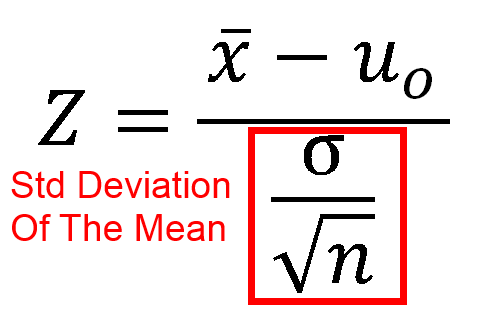Where

• x̄ is the sample mean
• u0 is the population mean
• σ           is the population standard deviation
• n is the test sample size

As you can see, the sigma divided by the square root of n that we saw as we measured the average roll of an increasing number of dice is a key part of the equation.

## 6 thoughts on “An Intuitive Guide To Statistical Significance”

1.B A Taylor

Very helpful! Btw, in my browser (Chrome) the 3rd std deviation percentage shows as 7 rather than 99.7.

2.Fairly Nerdy Post author

Thanks, fixed that error!

3.Brian

Is there a typo in the paragraph below or am I just really confused? Shouldn’t the last sentence be that 95% of the results fall withing 2 standard deviations?

68 percent of the data will fall within 1 standard deviation of the mean
95 percent of the data be within 2 standard deviations
99.7 percent of the data is within 3 standard deviations

So if we know the mean value and standard deviation of our data, we know the distribution of results that we expect to get. We know that if we get a result that is 2 standard deviations away from the mean, that it is a rare event, because 95% of the results will fall within 1 standard deviation of the mean.

Also, not to nitpick but I am glad to have found this page and don’t want you to lose any favor with readers because of some little mistakes. The apple weight section reads like it was done by voice-to-text with some close but not quite there typos. For instance, “weight a back of apples”. “Weight” is used several times and “weighed” is used in others. After one or two of these error, it makes me start to question the accuracy of the information that I am here to read. I doubt that that is the vibe that you’re trying to put out here.

Thanks for making this information approachable and the cheat sheet chart is just what I was looking for!

4.Fairly Nerdy Post author

Brian,

Thank you for notifying me about those errors. You were correct on all accounts, I have fixed those now

Edit – I tried to send you an email to the address that got logged in the wordpress comment field, however that email didn’t go through. If you come back to this page and see this, I owe you a favor for finding these errors and pointing them out, so if you ever need a favor that it makes sense for a stranger on the internet to provide, let me know

Scott

5.Burt

On the “Standard Deviation of Average Roll” graphs, should the x-axis be labeled “Number of Rolls”, rather than “Number of Dice”?

1.Fairly Nerdy Post author

Burt,

I would say that it is correct as is. For instance if you look at the chart labeled “Average Roll As You Increase The Number of Dice” (either chart) you can see that the shape of the curves change with different numbers of dice

The “Standard Deviation Of Average Roll” chart shows the standard deviation of that entire curve. I.e. if you were to take every point on that curve and take the standard deviation you would get that downward sloping curve.

Now, i’m sure someone could actually take the standard deviation of a continuous curve, but I cheated and just calculated all the possible outcomes of rolling 20 dice (there are 101 of them) in Excel and took the std deviation of that discrete set.

You can get that Excel file here, look at the “Rolling Dice” worksheet
http://www.fairlynerdy.com/Files/Cheat_Sheets/Hypothesis_Testing_Examples_Revision_1.xlsx

Note that there are other tabs associated with the Hypothesis testing book mentioned in this post which might not make sense when reading the Excel file standalone

I should note that your comment might also be correct, if what you meant was that you would roll a single die X times and sum those rolls, and take the Std deviation of every possible outcome, rather than X dice a single time. That statement would be interchangeable with my assertion